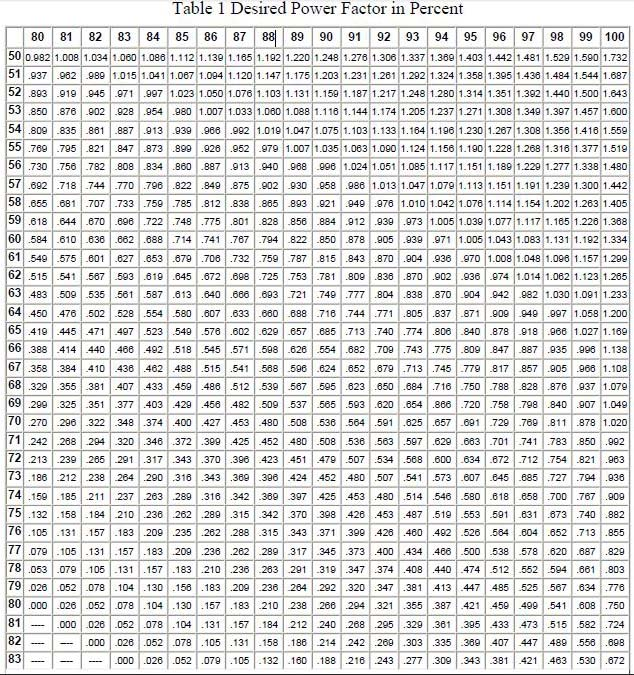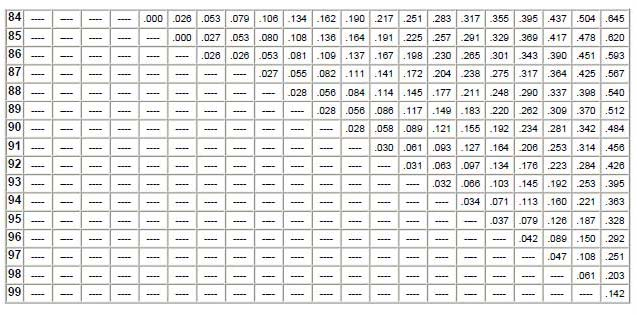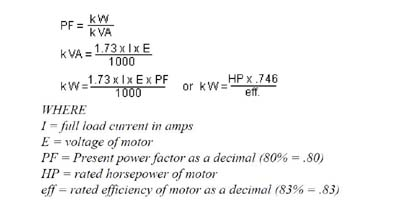# Capacitor sizing for power factor correction

Commonly method for power factor correction using the capacitor to generate reactive power ( kVAR ) to reduce apparent power ( kVA ) form inductive load.

This time i want share a simple method to sizing our capacitor to improve power factor for induction motor and utility electricity.This method also can use for capacitor bank sizing.

Power factor correction is the one of famous topic for all electrical person at around the world.Many discussion and debate about how to sizing the capacitor for power factor correction.

## How to sizing capacitor for power factor correction?

To properly sizing the amount of capacitor (kVAR) required to correct the lagging power factor,we  must have three (3)  important of information below:

• kW (kilowatts)
• Existing Power Factor ( % )
• Desired Power Factor ( % )

From this information,now we can calculate the capacitor size for power factor correction.The formula to calculate the required kVAR is:

#### Factor from Table 1 below x kW = kVAR of capacitors required.

Calculation Example :

1 unit air-compressor ( 3 phase 415 VAC ) used an average of 90 kW with an existing power factor of 80%.The desired power factor is 95%. The factor value for this case is 0.421 to raise the power factor from 80% to 95% using table 1.

0.421 x 90 kW = 38 kVAR

So we need capacitor size 38 kVAR to get power factor 95% for 1 unit air-compressor 90 kW.If working power (kW) or Present Power Factor are not known you can calculate from the following formulas to get the three basic pieces of information required to calculate kVAR.The best range for  desired power factor is around 95% until 100%.1.haitham hashim says

If I had known this site earlier when she entered the Faculty of Electrical Engineering, read the threads started by Mr. Admin and I become a better engineer, thank you, Mr. Adam

•lemau says

Thanks for ur compliment

•SANJAY says

2.Sinnadurai Sripadmanaban says

Are pf and efficiency of motors given nameplate of all motors?.
If no-load current is known(given in nameplate) can we estimate the size of capacitor required a) to start the motor (by reducing starting current)
b) to correct pf?.

•lemau says

We can estimate the full load ampere using the formula : – kW = ampere x Volt x 1.74

3.Pieface says

Hi
Nice explanation
There is something similar explained for capacitor sizing

•lemau says

hi pieface,thanks for share nice info :D

4.stefanb2hv says

hello, nice article!!! I am really impressed. Actually this is my first time reading your post. But I am very fascinated by the way you explained this simple method in sizing up a to improve power factor for induction motor and utility electricity. Thanks for sharing this information. Really good. I will be subscribing to your feed. Rest assured that I will read your future posts..

•lemau says

HI stefanb2hv..thanks for ur compliment…are u supplier from germany?i like germany stuff.it have a high quality :D

5.BHARGAV says

dear sir,
if i have a only motors HP ratting, then how i can know require KVA of capacitor??
thanking yoy

•lemau says

no problem bro,
do you mean for power factor correction for induction motor?
1) Convert hp to kW;formula : 1 hp = 0.746 kW
2) from kW,you convert to Ampere;formula : I = kW / volt x 1.73
3) from ampere you can find the kVAR for power factor capacitor..for more detail please read my post about capacitor sizing for power factor.

•Faisal says

1 kw= 1.34102 British horsepower 😶😶😶

6.جودة عبد الحليم السيد says

I need no. of power factor capstor for 11 Kw motor

7.جودة عبد الحليم السيد says

I need no. of power factor capstor for 11 Kw motor

8.ask says

My family members always say that I am killing my time here at web, except I know I
am getting experience all the time by reading such good articles.

9.asif says

dEAR SIR

There is panel with having a panel Incomer 800Amp now they want to install capacitor panel for same panel

How much Capacitor Kvar needed for the Panel

The method of calculation

10.Henryeio says

There is panel with having a panel Incomer 2600Amp now they want to install capacitor panel for same panel

How much Capacitor Kvar needed for the Panel

11.sadique says

Dear sir,
I considered 65% diversity factor of connected load then what will be initial power factor.
e.g.
c.l. =275 kw
div =65%
TMD =178.7 kw

12.Akshay Madankar says

How can i calculate the value of capacitor required for 2hp motor?

•blessy pauly says

hey dude,
1) Convert hp to kW; formula : 1 hp = 0.746 kW
For ur motor..2HP motor=1.492KW
After this, calculate capacitor size with formula given above in the article .
for tat u have to known either voltage or efficiency.

13.ASIF says

during design of LT system initailey we dont ware of existing power of the system then in that case how we can caluculate capatior bank size : Suppose load of the plat is 800kw (Lighting power +havc +utility load) how much capacitor bank will required for unity PF

14.spec says

15.Rabiu Abdullahi muhammad says